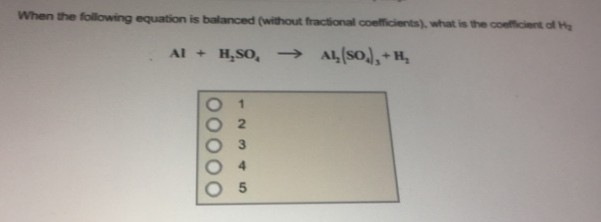# Problem: When the following equation is balanced (without fractional coefficients), what is the coefficient of H2?Al + H2SO4 → Al2(SO4)3 + H2

###### FREE Expert Solution
83% (359 ratings)###### Problem Details

When the following equation is balanced (without fractional coefficients), what is the coefficient of H2?

Al + H2SO4 → Al2(SO4)3 + H2